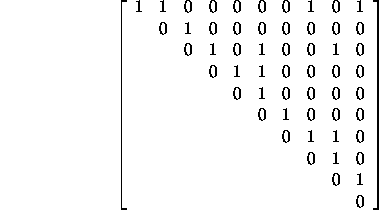CSE 5311 Section 501 Fall 1998
Homework 5
Due: April 6, 1998, in class

1. List the vertices in each connected component after each iteration of the second loop in the Connected-Components code when applied to graph G. Graph G = (V, E), where V = {a, b, c, d, e, f, g, h, i, j} and edges in E = {(a,c), (a,b), (e,f), (h,i), (e,g), (a,d), (e,d), (b,d), (c,a), (h,j)} are processed in the order listed.
2. Argue on the basis of Theorem 22.1 in the textbook that we can obtain amortized time bounds of O(1) for Make-Set and Find-Set and O(lg n) for Union using the linked-list representation and the weighted-union heuristic.
3. Consider the following adjacency matrix for an undirected graph:The 1s here indicate the presence of an edge. I want you to construct your own set of weights for each edge. To make sure you all have unique weights, use the non-zero digits from your Social Security Number, your birthday, and your phone number as weights, in that order (with repetition if necessary).

Show the minimum spanning tree that results from using Kruskal's algorithm and from using Prim's algorithm. Show each step of the procedure for both algorithms.

4. Show the order in which the nodes in graph G are visited (dequeued) using a Depth-First Search and a Breadth-First Search. G = {a, b, c, d, e, f, g} and E = {(a,b), (a,c), (b,d), (c,e), (d,f), (e,f), (e,g)}. Start at node a and check edges in alphabetical order.
5. Give a topological sort for the following relations: (a < b, a < c, d < b, e < c, a < e, a < d).
6. Implement the MST-Prim algorithm described in the textbook using a priority queue. You can use this code to generate a solution for problem 3 in this homework set, if you wish. Send all code, sample files, and compile information to cs5311-turnin@cse.